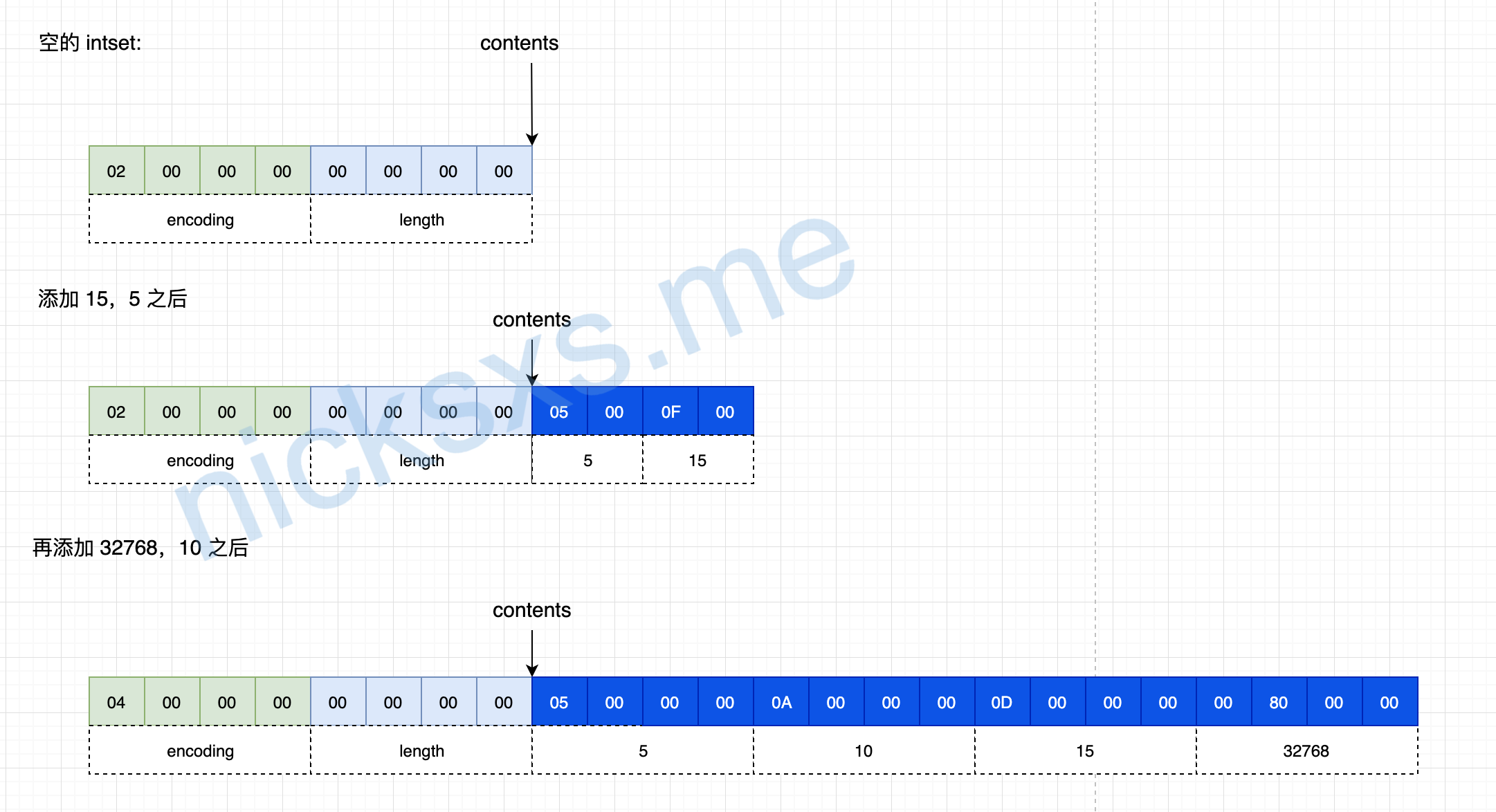# redis 数据结构介绍三 - 第三部分 整数集合Nick

redis中对于 set 其实有两种处理，对于元素均为整型，并且元素数目较少时，使用 intset 作为底层数据结构，否则使用 dict 作为底层数据结构，先看一下代码先

typedef struct intset {    // 编码方式    uint32t encoding;    // 集合包含的元素数量    uint32t length;    // 保存元素的数组    int8t contents[];} intset;/ Note that these encodings are ordered, so:  INTSETENCINT16 < INTSETENCINT32 < INTSETENCINT64. */#define INTSETENCINT16 (sizeof(int16t))#define INTSETENCINT32 (sizeof(int32t))#define INTSETENCINT64 (sizeof(int64t))

>Flexible array members(https://en.wikipedia.org/wiki/Flexiblearraymember#citenote-1) were introduced in the [C99](https://en.wikipedia.org/wiki/C99) standard of the [C programming language](https://en.wikipedia.org/wiki/C(programminglanguage)) (in particular, in section §6.7.2.1, item 16, page 103).(https://en.wikipedia.org/wiki/Flexiblearraymember#citenote-2) It is a member of a struct, which is an array without a given dimension. It must be the last member of such a struct and it must be accompanied by at least one other member, as in the following example:

struct vectord {    size_t len;    double arr[]; // the flexible array member must be last};﻿

﻿## 评论